# Band Structures and Newton's Method¶

### Example – Quantum Mechanics¶

Last edited: April 15 2016

One of the first great triumphs of quantum mechanics in applied physics was the electron band theory of solids . The band structure arises due to the periodic potential experienced by the electrons in a solid. In the following, we will use Newton's method to calculate the band structure for the simple Dirac comb potential in one dimension.

### Particle in a One-Dimensional Lattice¶

A simple model for a particle in a periodic lattice, i.e. a crystal, is a periodic potential of delta peaks in one dimension, also known as the Dirac comb potential,

$$V(x) = V_0 \sum_{j}\delta(x-ja),$$

where $a$ is the distance between the delta function peaks. In order to make this seem like a more realistic model, we can imagine that the potential wraps around in a circle that includes a macroscopic amount of peaks, $N \sim 10^{23}$. This means that the wavefunction of the system has to fulfill periodic boundary conditions,

$$\psi(x+Na)=\psi(x).$$

### Bloch's Theorem¶

Bloch's theorem  states that for a periodic potential

$$V(x + a ) = V(x)$$

of period $a$, the wavefunction $\psi(x)$ satisfies

$$\psi(x +a) = e^{iKa} \psi(x).$$

Using periodic boundary conditions as stated above, we find

$$\psi(x+Na) = e^{iNKa} \psi(x) = \psi(x).$$

Hence, we must have $e^{iKNa} = 1$ which means

$$K=\frac{2\pi n}{Na}, \qquad n\in \mathbb{Z}.$$

For large $N$, which is the case in macroscopic materials, these values of $K$ essentially represent a continuum.

The above implies that one only needs to find $\psi(x)$ within a single period with respect to $x$, for example $0<x<a$. Bloch's theorem then determines $\psi(x)$ in the next period of the crystal and so forth.

### Solving the Schrödinger Equation¶

The time-independent Schrödinger equation (SE) in one dimension reads

$$-\frac{\hbar^2}{2m} \frac{d^2}{dx^2}\psi(x) + V(x)\psi(x) = E\psi(x).$$

For $0<x<a$, $V(x)$ = 0 which yields the general solution

$$\psi(x) = A\sin(kx) + B\cos(kx), \qquad(0<x<a)$$

where

$$k=\frac{\sqrt{2mE}}{\hbar}.$$

Bloch's theorem then gives $\psi(x)$ for $-a$ < $x$ < 0,

$$\psi(x) = e^{-iKa}[A\sin k(x+a) + B\cos k(x+a)], \qquad(-a<x<0).$$

The wavefunction must be continous at $x=$ 0. This yields the condition

$$B = e^{-iKa}[A\sin(ka) + B \cos(ka)].$$

The derivative of the wavefunction is, however, not continuous at $x=$ 0, due to the delta functions in the potential. Let us derive the condition that the derivative of the wavefunction must fulfill. With a potential

$$V_\delta(x) = \alpha \delta(x),$$

$$-\frac{\hbar^2}{2m}\psi''(x) + \alpha \delta(x) \psi(x) = E\psi(x),$$

where $\psi''(x)$ denotes the second spatial derivative of the wavefunction. We rearrange the above equation and integrate over $x$, from $-\epsilon$ to $\epsilon$,

$$\int_{-\epsilon}^{\epsilon}\frac{\mathrm{d}\psi'(x)}{\mathrm{d}x} \mathrm{d}x= \int_{-\epsilon}^{\epsilon} \frac{2m}{\hbar^2}[\alpha\delta(x) -E]\psi(x)\mathrm{d}x.$$

With the concept of distributions, this equals

$$\psi'(\epsilon) - \psi'(-\epsilon)= \frac{2m}{\hbar^2}\alpha\psi(0) -\int_{-\epsilon}^{\epsilon} \frac{2m}{\hbar^2}E\psi(x)\mathrm{d}x.$$

In the limit $\epsilon \rightarrow$ 0, we get

$$\psi'(0^+)-\psi'(0^-) = \frac{2m}{\hbar^2}\alpha\psi(0).$$

With our notation above, this is equivalent to

$$kA - ke^{-iKa}[A\cos(ka) - B\sin (ka)] = \frac{2m V_0}{\hbar^2} B.$$

### Forbidden Energies¶

Using the conditions for $\psi$ and $\psi'$ above, we can eliminate $A$ and $B$ to obtain a condition for the values of $k$:

$$\cos(Ka) = \cos(ka) + \frac{mV_0}{\hbar^2k} \sin(ka).$$

Since $\|\cos(Ka)\| \leq 1$, certain values of $k$, and consequently also values of the energy $E$, are forbidden. Why? Because the magnitude of the right-hand side can at most be one for the equation to have a solution. Remember, we assume that $K$ forms a continuum. This means that as long as the magnitude of the right-hand side is at most one, we can find a $K$ that solves the equation.

To simplify calculations, we introduce the dimensionless parameters

$$z \equiv ka$$

and

$$\beta \equiv \frac{maV_0}{\hbar^2}$$

so that the right-hand side of the above equation becomes

$$f(z) = \cos(z) + \beta \frac{\sin(z)}{z}.$$

(For a more in-depth derivation of this function, see  or .)

Let's now look at how $f(z)$ behaves by plotting it.

In :
# Inline plotting:
%matplotlib inline
# Import needed modules:
from __future__ import division
import numpy as np
from numpy import pi
import matplotlib.pyplot as plt
import matplotlib.collections as collections

# Set common figure parameters:
newparams = {'axes.labelsize': 14, 'axes.linewidth': 1, 'savefig.dpi': 300,
'lines.linewidth': 1.0, 'figure.figsize': (8, 3),
'figure.subplot.wspace': 0.4,
'ytick.labelsize': 10, 'xtick.labelsize': 10,
'legend.fontsize': 10, 'legend.frameon': False,
'legend.handlelength': 1.5}
plt.rcParams.update(newparams)

# Define default values of the parameters beta, and start and stop values of z.
DEFAULT_BETA = 10
DEFAULT_ZSTART = 0
DEFAULT_ZSTOP = 20

# Define needed functions
def f(z, beta=DEFAULT_BETA):
"""Returns function value f(z) for a given value of beta."""
return np.cos(z) + beta*np.sin(z)/z

def plot_f(beta=DEFAULT_BETA, zstop=DEFAULT_ZSTOP, zstart=DEFAULT_ZSTART, ax=None):
"""
Plots f(z) for a given beta and range of z.
"""
if ax is None:
ax = plt.gca()

z = np.delete(np.linspace(zstart, zstop, 10000), 0)
ax.set_xlabel(r'$z$')
ax.set_ylabel(r'$f(z)$')
pi_start = zstart//pi
pi_stop = zstop//pi
ticks = np.arange(pi_start, pi_stop+1)
ax.set_xticks(ticks*pi)
fvalues = f(z, beta)
ticklabel = ['%d $\pi$' % t if t!=0 else'0' for t in ticks]
ax.set_xticklabels(ticklabel)
ax.plot(z, fvalues, label=r'$\beta = {}$'.format(beta))

def plot_allowed_area(zstop=20, zstart=0, ax=None):
"""Plot horizontal lines at y=+/- 1"""
if ax is None:
ax = plt.gca()
collection=collections.BrokenBarHCollection(xranges=[(zstart,zstop-zstart)],yrange=(-1,2), facecolor='#cccccc' )

# Plot f(z) with beta equal to 5:
plot_f(beta=5.0)
plot_allowed_area()
plt.legend()
plt.grid()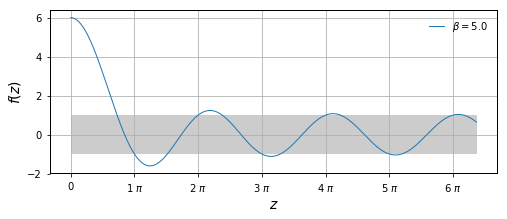The above plot shows $f(z)$ for $\beta=5$ together with a grey area, indicating the allowed values of $f(z)$. The values of $z$ for which the graph is outside the grey area, correspond to forbidden energies, or band gaps. Likewise, we find bands consisting of permissible energy values and separated by band gaps.

We want to calculate the values $z$ for which we have $f(z) \in [-1,1].$ An interesting feature to note about $f(z)$ is that if $z$ is an integer multiple of $\pi$, meaning $z=n\pi , (n\in \mathbb{Z})$, then $f(z)$ assumes values that are independent of $\beta$: $$f(n\pi) = (-1)^n .$$ This marks the upper bound of a band. Hence, we can focus on finding the lower bounds of $z$ for each band. This will be done using Newton's Method.

### Newton's Method¶

Newton's method is an iterative method for finding the roots of a function by following its tangent line from an initial guess.

In our case, we want to find out when

$$f(z) - y_0 = 0,$$

given $y_0 = \pm 1$, where the sign depends on which band we consider.

Given an initial guess for a value $z$ of the root, Newton's method computes the next, hopefully better, approximation of the correct value, according to

$$z_{n+1} = z_n - \frac{f(z_n) - y_0}{f'(z_n)}.$$

In the case of our function

$$f(z) = \cos(z) + \beta \frac{\sin(z)}{z},$$

we have

$$f'(z) = -\sin(z) + \beta \frac{z\cos(z) - \sin(z)}{z^2}.$$

The derivative, $f'(z)$, and a function returning the next Newton iteration, $z_{n+1}$, are implemented below.

In :
def df(z, beta=DEFAULT_BETA):
"""Returns the derivative of f(z)."""
return -np.sin(z) + beta*(z*np.cos(z) - np.sin(z))/z**2

def newton_iteration(z_n, beta=DEFAULT_BETA, y0=1):
"""One iteration of Newton's method with function f.
For a given guess of root value, z_n, it returns z_(n+1).
"""
return z_n - (f(z_n, beta) - y0)/df(z_n, beta)


### Testing Newton's Method¶

To check whether the method works as expected, we can test it by trying to locate the start value of $z$ for different bands when starting at the end value, a integer multiple of $\pi$. The following code will run $N$ iterations of Newton's method with given initial values $z_0$, and plot the points calculated at each iteration.

In :
def test_newton_iterations(z0, beta=DEFAULT_BETA, y0=1, N=5):
"""Returns the N first iterations of the Newton's method for f(z) given intial value z0."""
zn = np.zeros(N+1)
zn = z0
for i in range(N):
zn[i+1] = newton_iteration(zn[i], beta, y0)
return zn

def insert_annotations(zn, values, ax=None):
"""Code for putting labels near the iteration points
Arguments:
zn        Iteration points
values    Function values f(z) at points zn
ax        Axes instance
"""
if ax is None:
ax = plt.gca()
previous_ytext = 0
previous_z = 10e9
for i,z in enumerate(zn):
if abs(z-previous_z) < 0.3:
ytext = previous_ytext + 10
else:
ytext = 4
ax.annotate("z{}".format(i), xy=(z,values[i]), textcoords='offset points', xytext=(0, ytext))
previous_ytext = ytext
previous_z = z

def plot_tangents(zlist, beta=DEFAULT_BETA, ax=None):
"""Plot the tangents of f(z) on the points in zlist."""
if ax is None:
ax = plt.gca()
zstart, zstop = ax.get_xlim()
z = np.linspace(zstart, zstop, 1000)
for zn in zlist:
y = df(zn, beta)*(z - zn) + f(zn, beta)
ax.plot(z, y)

def purge_last_equal_iterations(iterations, precision=0.005):
"""Remove the last points that are practically equal"""
ind = np.where(np.abs( iterations - iterations[-1] )< precision)
return iterations[:(ind+1)]

def scale_axis_pi(ax=None):
"""Changes the x-axis to show ticks on integer values of pi."""
if ax is None:
ax = plt.gca()
zstart, zstop = ax.get_xlim()
pi_start = zstart//pi
pi_stop = zstop//pi
ticks = np.arange(pi_start, pi_stop+1)
ax.set_xticks(ticks*pi)
ticklabel = [r'%d $\pi$' % t if t!=0 else'0' for t in ticks]
ax.set_xticklabels(ticklabel)

In :
beta = 7
z0_list = np.array([1,2,3])

for i, z0 in enumerate(z0_list):
y0 = -1 if z0%2==0 else 1
plt.figure()

iterations = test_newton_iterations((z0)*pi, beta=beta, y0=y0, N=5)
iterations = purge_last_equal_iterations(iterations)
values = f(iterations, beta)

plot_f(zstop=5.5*pi, beta=beta)
plt.plot(iterations, values, '*')
insert_annotations(iterations, values)

scale_axis_pi()
plot_allowed_area()
plot_tangents(iterations[:1], beta)

plt.ylim([-4,12])
plt.xlim([0,5.5*pi])
plt.title(r'$z_0={}\pi$, $\beta={}$, $y={}$'.format(z0, beta, y0))
plt.grid()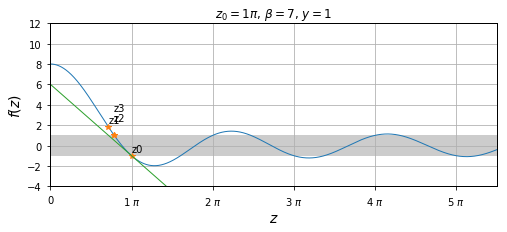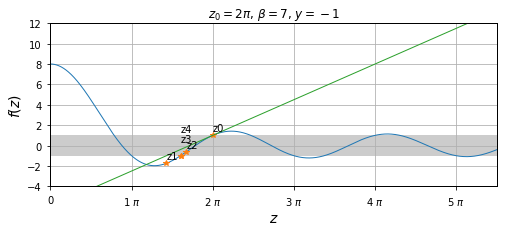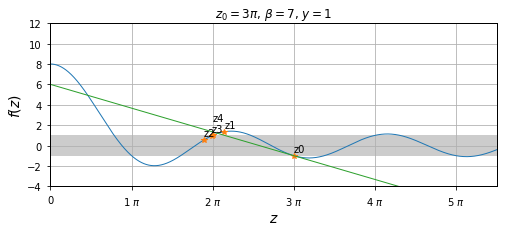From the above plots, one can see that the convergence of Newton's method is highly dependent on the initial guess, $z_0$. For example, in the last plot we see that Newton's method converges towards the end value $z=2\pi$ of the previous band instead of the start value of the current band. To obtain the desired convergence, it is crucial to avoid undesired stationary points.

The below function is implemented in order to run Newton's method up to a given precision.

In :
PRECISION = 0.000001
MAX_NEWTON_ITERATIONS = 30 # Making sure we don't get an infinite loop.

def run_newton(z_start, y0, beta=DEFAULT_BETA):
"""Runs Newton's method to calculate the lower value z with a given precision."""
z_new = z_start
iteration = 0
while(abs(f(z_new, beta) - y0) > PRECISION):
iteration += 1
assert iteration <= MAX_NEWTON_ITERATIONS, "The method has run for max iterations={}".format(MAX_NEWTON_ITERATIONS)
z_new = newton_iteration(z_new, beta, y0)
return z_new


This function will be used to calculate the lower bound of each of the first ten bands. Since we know the end value of each band, it might be a good idea to begin the search from values close to, but slightly smaller than those end values. This is what the following code does, including a plot of the final band structure.

In :
def calculate_bands(beta=DEFAULT_BETA, num_bands=10, start_band=0):
"""Returns the z-values of the beginning and end of each band.

The values are formatted as a 2xN matrix where N is the number of bands.
"""
band_numbers = np.arange(start_band, num_bands+start_band)
end_points = (band_numbers+1)*pi # The known end points
start_points = np.zeros(num_bands) # Initializing the array of start points
previous_end_point = 0

# Loop through each band and find the start point of the band
# by guessing a value close to the end point.
for n in range(num_bands):
band_number = band_numbers[n]
end_point = end_points[n]
z0 = end_point - 0.2*pi # Initial guess
y0 = (-1)**(band_number) # The value of f(z) we are looking for.

# Find new possible start_point
possible_start_point = run_newton(z0, y0, beta)
# If not between end points try a new initial guess z0
while(not (previous_end_point < possible_start_point < end_point) ):
z0 -= pi*0.1
assert (z0 > previous_end_point) # Make sure the initial guess is between end points
possible_start_point = run_newton(z0, y0, beta)

start_points[n] = possible_start_point
previous_end_point = end_point

# Assert that all end points comes after the start_points
assert (end_points > start_points).all(), "Not all end points are after start points"
return np.array([start_points, end_points])


Using the above function, the width of the energy bands are calculated.

In :
b = calculate_bands(start_band=2)
print('Band widths:\n', b-b)

Band widths:
[ 1.35764238  1.65766308  1.88877155  2.0668647   2.20564343  2.31548818
2.40389076  2.47619049  2.53620199  2.58668506]


The following code will plot the energy bands for a given $\beta$.

In :
def plot_bands(bands, ax=None):
"""Plots the bands given a list of tuples containing beginning and end positions of the band."""
if ax is None:
ax=plt.gca()
xranges = zip(bands, bands-bands)
ylim = ax.get_ylim()
collection=collections.BrokenBarHCollection(xranges=xranges, yrange=ylim, facecolor='#bb9999')
return ax

beta = DEFAULT_BETA
zstop = 30
num_bands= int(zstop//pi)

bands = calculate_bands(beta=beta, num_bands=num_bands)
plot_f(beta=beta, zstop=zstop)
plot_bands(bands)
plt.ylim([-2,2])
plt.title(r"Energy bands for $\beta=$ %d " % beta);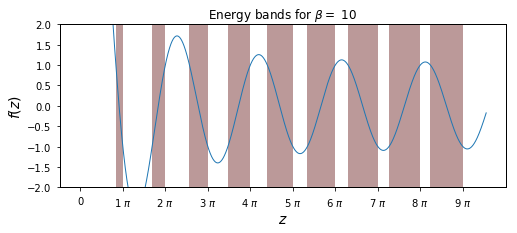As we see, the band widths increase with increasing $z$. Let's calculate the lowest allowed energy value:

In :
print(r'Lowest allowed value of z = %.4f.' % bands[0,0])

Lowest allowed value of z = 2.6277.


We know that $z$ is related to $k$ by

$$z=ka,$$

and that $k$ is related to the energy $E$ by

$$k = \frac{\sqrt{2mE}}{\hbar}.$$

Setting $\hbar=m=a=1$ for simplicity, we obtain

$$E = \frac{z^2}{2}.$$

Hence, the lowest allowed electron energy in the crystal is $E_0 = 3.5703$. In this case $\beta=10$, which corresponds to a delta function strength $V_0=10$. Similarly, one can calculate the range of allowed energies in each band.

It is also interesting to investigate how the band widths depend on $\beta$.

In :
def plot_bandwidths(betas, num_bands):
"""Plots the bandwidths against band_number for different betas."""

for beta in betas:
bands = calculate_bands(beta, num_bands)
width = bands[1,:] - bands[0,:]
plt.plot(width, label=str(beta))
plt.title(r'Band widths for different values of $\beta$')
plt.xlabel('Band number')
plt.ylabel('Band width')
plt.legend(loc=4)

plot_bandwidths(betas=[5,10,20,30,40,50], num_bands=60)
plt.plot([0,60],[pi, pi],':');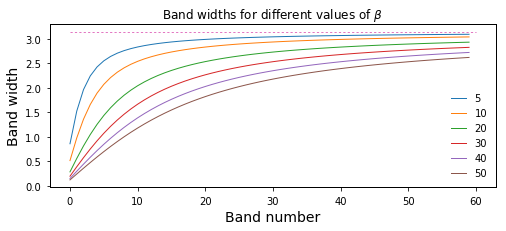We see that the width of the bands increases towards $\pi$. When the band width is equal to $\pi$, it means that the bands are in fact connected and can be regarded as one continuous band.

## References:¶

: Hemmer, P. C. Kvantemekanikk. Tapir Akademisk Forlag, 2005
: Griffiths, D. J. Introduction to Quantum Mechanics. Pearson Education, 2004.